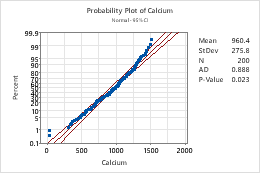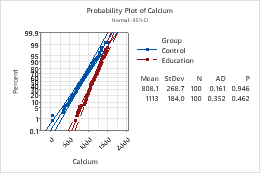# Select a probability plot

Choose Graph > Probability Plot, then select a probability plot based on how you want the plot to look.

## Single

Create a probability plot that displays the distribution of values for one variable. For example, the following probability plot shows the distribution of calcium values.